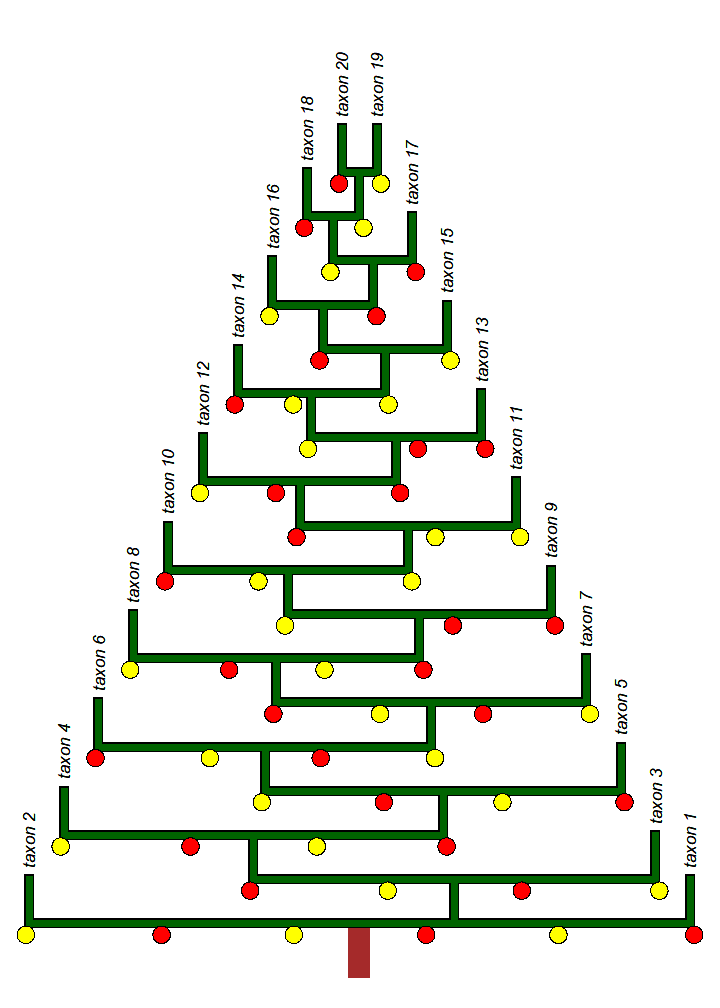## Monday, December 17, 2018

### A phylogenetic Christmas tree in R (adapted from Gustavo Ballen)

Today, a colleague Gustavo Ballen shared an cleverly decorated phylogenetic Christmas tree that he created using the phylogenetic visualization software, FigTree.

I hope that he doesn't mind that I'm sharing a script to recreate his plot in R:

``````library(phytools)
library(plotrix)
library(phangorn)
tree<-stree(20,"left")
tree\$tip.label<-paste("taxon_",1:20,sep="")
for(i in 1:tree\$Nnode) if(i%%2==1) tree<-rotate(tree,Ntip(tree)+i)
tree\$edge.length<-c(0,rep(1,nrow(tree\$edge)-1))
tree<-di2multi(tree)
plot.new()
par(mar=rep(0.1,4))
plot.window(xlim=c(0.5,20.5),ylim=c(-1,20))
lines(c(10.5,10.5),c(-1,-0.2),lwd=15,col="brown",lend=2)
plotTree(tree,direction="upwards",ftype="i",fsize=0.7,offset=0.5,
plotTree(tree,direction="upwards",ftype="i",fsize=0.7,offset=0.5,
lastPP<-get("last_plot.phylo",envir=.PlotPhyloEnv)
for(i in 1:tree\$Nnode){
node<-i+Ntip(tree)
daughters<-Children(tree,node)
daughters<-daughters[c(1,length(daughters))]
xx<-lastPP\$xx[daughters]
yy<-rep(lastPP\$yy[node],2)
cols<-if(i%in%c(2,5,6,9,10,13,14,17,18)) c("red","yellow") else
c("yellow","red")
draw.circle(xx-0.1,yy-0.25,0.25,col=cols)
draw.circle(xx+0.1,yy-0.25,0.25,col=cols)
length.out<-floor(diff(xx)/4)+2
if(length.out>2){
xx<-seq(xx,xx,length.out=length.out)
if(length(xx)==3) xx<-if(xx>mean(range(lastPP\$xx)))
xx+0.25*(xx-xx) else xx-0.25*(xx-xx)
xx<-xx[2:(length(xx)-1)]
cols<-if(i%in%c(2,5,6,9,10,13,14,17,18)) rep(c("yellow","red"),
ceiling(length(xx)/2)) else rep(c("red","yellow"),
ceiling(length(xx)/2))
for(j in 1:length(xx)) draw.circle(xx[j],yy-0.25,0.25,
col=cols[j])
}
}
``````Happy holidays!

#### 1 comment:

1.Note: due to the very large amount of spam, all comments are now automatically submitted for moderation.